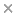BaseSpace
Correlation Engine 2.0Search sequence regions

###### filter terms:
Sizes of these terms reflect their relevance to your search.

Effective photon energy absorption (EABF(eff)) and exposure buildup factors (EBF(eff)) have been calculated based on the effective energy concept, for some dosimetric materials such as water, polymethyl methacrylate (PMMA), polystyrene, solid water (WT1), RW3 (Goettingen Water 3), and ABS (acrylonitrile butadiene styrene), for MV X-rays and (60)Co gamma rays. Firstly, the equivalent atomic numbers (Z(eq)) of the given materials have been determined using the effective photon energies (E eff). Then, the five-parameter geometric progression (G-P) fitting approximation has been used to calculate both EABF(eff) and EBF(eff) values. Since the G-P fitting parameters are not available for the E eff values of the given materials, a linear interpolation in which a function of the logarithm of the variable is used has been performed, in order to calculate the parameters in each E eff, which will be further used for the determination of EABF(eff) and EBF(eff). In the present paper, water equivalence properties of the given materials are also discussed based on the effective buildup factors. In this study, special emphasis is placed on the calculation of EABF(eff) and EBF(eff) values of different materials for photons that are not monoenergetic but heterogeneous in energy, to obtain an initial and prior knowledge of the probable energy and buildup of photons at locations of interest, i.e., to understand whether the real absorbed dose occurs at the surface or somewhere inside the medium of interest.

### Citation

Murat Kurudirek. Photon buildup factors in some dosimetric materials for heterogeneous radiation sources. Radiation and environmental biophysics. 2014 Mar;53(1):175-85

PMID: 24287785

View Full Text